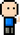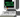# Josh Meets Computer# Matplotlib Histogram Animation

February 4, 2018

## Using Matplotlib path patch to animate rectangles

Techniques for animating a histogram by changing the vertices of each bin of the histogram — treating the bars of the graph as drawn rectangles.

``````import numpy as np
import matplotlib.pyplot as plt
import matplotlib.patches as patches
import matplotlib.path as path
import matplotlib.animation as animation
from matplotlib import rc
from IPython.display import HTML
%matplotlib inline

rc('animation', html='html5')  # sets animation display from none to html5``````
``````# create histogram
np.random.seed(7)
data = np.random.randn(1000)
num_bins = 20

freq, bin_edges = np.histogram(data, num_bins)``````

### Getting the corners of the rectangles

Bin_edges is an array of the box positions from left to right.

The first index of bin_edges would be the left most bin left edge position, the second index is the left most bin right edge position. The left edges index from 0, right edges index from 1.

``````left = np.array(bin_edges[:-1])
right = np.array(bin_edges[1:])
bottom = np.zeros(num_bins)
top = bottom + freq

assert len(left) == num_bins``````

For each rectangle we need one ‘MOVE TO’ path, three ‘LINE TO’ paths and one ‘CLOSE POLY’ path. One array, called verts, will hold all the vertices (coordinates) of the corners of each histogram rectangle. There will be another array of the same length that will hold the codes of what to do with these vertices. The first vertice will have a move to command, the next three will have a line to command, and the last one will have the close poly command.

``````num_verts = num_bins * (1 + 3 + 1)
verts = np.zeros((num_verts, 2)) #(x, y) coordinates for all the verts
# left wall -- vertex 1 and vertex 2
verts[0::5, 0] = left
verts[0::5, 1] = bottom
verts[1::5, 0] = left
verts[1::5, 1] = top

# right wall -- vertex 3 and vertex 4
verts[2::5, 0] = right
verts[2::5, 1] = top
verts[3::5, 0] = right
verts[3::5, 1] = bottom

codes = np.ones(num_verts, int) * path.Path.LINETO
codes[0::5] = path.Path.MOVETO
codes[4::5] = path.Path.CLOSEPOLY``````

### Create an animation function

The animation function updates the location of the four vertices based on incoming data. In this example only the frequency of each bin changes

``````patch = None  # this will be a patch object later

def animate(i):
"""
New data updates the frequency of each bin.
For example, if you have a uniformly scaled dataset
you can see the spread of each feature.
"""

data = np.random.randn(1000)
freq_values, bins = np.histogram(data, num_bins)
bottom = np.zeros(num_bins)
top = bottom + freq_values

verts[1::5, 1] = top
verts[2::5, 1] = top

return [patch,]    ``````

## Create plot, patches and animation

Create a series of lines from the vertice values, assign the first one vertice with the ‘MOVE TO’ (moves the drawing pen) and the last vertice with the CLOSEPOLY code (draws a line segment to the starting vertice).

Take these series of paths and create a PathPatch of many poly curves to add to the subplot.

``````fig, ax = plt.subplots();
bar_path = path.Path(verts, codes);

patch = patches.PathPatch(bar_path, facecolor='blue', edgecolor='yellow', alpha=0.5);

``````ani = animation.FuncAnimation(fig, animate, 10, blit=True, repeat=True);